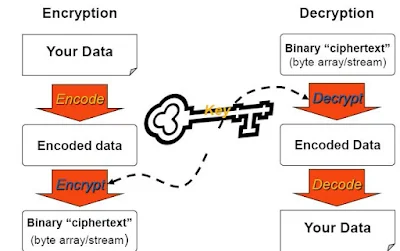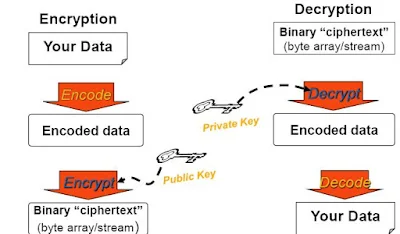# How to encrypt/decrypt data in asp.net using Symmetric algorithm

In this post, I am going to show you how to encrypt and decrypt data in ASP.NET using a symmetric algorithm. Before we dive into the implementation details, let’s discuss some security-related terms.

## What is Hashing?

Hashing is a one-way algorithm that generates a secure fingerprint of data. Once the data is hashed, it cannot be recovered. It is commonly used to verify the integrity of downloaded files.

## What is Encryption?

Encryption is a two-way process used to secure data. It involves transforming data using an encryption algorithm and a key. The same key is used to encrypt and decrypt the data.

## Symmetric Encryption

Symmetric encryption uses the same key for both encryption and decryption. The key is used to transform the data, and it must be kept secure. Examples of symmetric algorithms include DES, Triple DES, RC2, and AES.## Asymmetric Encryption

Asymmetric encryption uses a related key pair: a public key and a private key. Data encrypted with a public key can only be decrypted with the corresponding private key, and vice versa. RSA is a popular asymmetric algorithm.## Steps for Encryption

To encrypt data symmetrically, follow these steps:

1. Choose an encryption algorithm.
2. Create or retrieve a key.
3. Generate an Initialization Vector (IV).
4. Convert the clear text data to an array of bytes.
5. Encrypt the byte array.
6. Store the encrypted data and the IV.

## Steps for Decryption

To decrypt the encrypted data, follow these steps:

1. Choose the same algorithm that was used for encryption.
2. Retrieve the key that was used.
3. Retrieve the IV that was used.
4. Retrieve the encrypted data.
5. Decrypt the data.
6. Convert the decrypted data back to its original format.

## Implementation

For this demonstration, I am going to use the Triple DES algorithm.

1. Generate the IV and Key:

``````static void Main(string[] args)
{
DESCryptoServiceProvider des = new DESCryptoServiceProvider();
des.GenerateIV();
des.GenerateKey();
Console.WriteLine("Key: {0}", String.Join(",", des.Key));
Console.WriteLine("IV: {0}", String.Join(",", des.IV));
}
``````
2. Create an `EncryptionManager` class with the following methods:

``````public class EncryptionManager
{
private byte[] Key = { /* Key bytes here */ };
private byte[] IV = { /* IV bytes here */ };

public string Encrypt(string inputString)
{
byte[] buffer = Encoding.ASCII.GetBytes(inputString);
TripleDESCryptoServiceProvider tripleDes = new TripleDESCryptoServiceProvider()
{
Key = Key,
IV = IV
};
ICryptoTransform ITransform = tripleDes.CreateEncryptor();
return Convert.ToBase64String(ITransform.TransformFinalBlock(buffer, 0, buffer.Length));
}

public string Decrypt(string inputString)
{
byte[] buffer = Convert.FromBase64String(inputString);
TripleDESCryptoServiceProvider tripleDes = new TripleDESCryptoServiceProvider()
{
Key = Key,
IV = IV
};
ICryptoTransform ITransform = tripleDes.CreateDecryptor();
return Encoding.ASCII.GetString(ITransform.TransformFinalBlock(buffer, 0, buffer.Length));
}
}
``````
1. Create a webpage with the following code:
``````<form id="form1" runat="server">
<tr>
<td>
<asp:TextBox ID="txtData" runat="server" TextMode="MultiLine"></asp:TextBox>
</td>
<td>
<asp:TextBox runat="server" ID="txtEncrypt" TextMode="MultiLine"></asp:TextBox>
</td>
<td>
<asp:TextBox runat="server" ID="txtDecrypt" TextMode="MultiLine"></asp:TextBox>
</td>
</tr>
<tr>
<td>
<asp:Button ID="btnEncrypt" runat="server" OnClick="btnEncrypt_Click" Text="Encrypt" />
<asp:Button ID="btnDecrypt" runat="server" Text="Decrypt" OnClick="btnDecrypt_Click" />
</td>
</tr>
</table>
</form>
``````
``````using System;
using System.Security.Cryptography;
using System.Text;

public partial class EncryptDecrypt : System.Web.UI.Page
{
EncryptionManager manager = new EncryptionManager();

protected void btnEncrypt_Click(object sender, EventArgs e)
{
txtEncrypt.Text = manager.Encrypt(txtData.Text);
}

protected void btnDecrypt_Click(object sender, EventArgs e)
{
txtDecrypt.Text = manager.Decrypt(txtEncrypt.Text);
}
}
``````

That’s it! You now have a basic understanding of symmetric encryption and how to implement it in ASP.NET using the Triple DES algorithm. Feel free to explore other encryption algorithms and enhance the implementation according to your needs.

Please note that the above code snippets are provided as an example and may need to be adjusted or modified to fit your specific requirements.